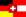Seite auf deutsch

# Cocktail Shaker Sort

In the following, we see how the primitive approach of BubbleSort can be considerably improved, by adding a few simple twists:

Legend:
pink: greater value in current comparison
red: smaller value in current comparison
blue: the part that is already sorted

We added two twists to the basic BubbleSort routine:

• In each round ("bubble up"), we remember whether we still found any two elements that needed to be swapped -> swapped = = false?
If not, the list is already sorted -> break.
• After each round from bottom to top ("bubble up") we make a round from top to bottom ("bubble down").
The result is the so-called "Cocktail Shaker Sort" (resp. "improved" or "bidirectional BubbleSort"). Its running time is still quadratic in the worst case; but in the best case it attains linear running time.

In the following, we show that the worst-case running time is still quadratic. The worst case happens (as with BubbleSort) when we are given an inversely sorted array1, that is, arr=(n,n-1,...3,2,1). After n-1 comparisons and n-1 swaps the first "bubble up" round is finished. The element n has arrived at its final position. The first "bubble down" round takes another n-1 comparisons and n-1 swaps. Then the element 1 has arrived at its final position. The remaining n-2 elements are still in reverse order, and each element has been moved back to its initial position. After one "bubble up" round and one "bubble down" round, the variables "start" is increased by 1 and "ende" is decreased by 1. The subarray considered by Cocktail Shaker Sort from now on is shrunk by 2 elements, and is of length n-2.

Letting V(n) denote the number of comparisons carried out by Cocktail Shaker Sort on an inversely sorted array, we obtain:
V(1) = 0
V(2) = 1
For n > 1 :
V(n) = (n-1) + (n-2) + V(n-2)
= n-1 + n-2 + n-3 + n-4 + V(n-4)
= n-1 + n-2 + n-3 + n-4 + ... + n-(n-2) + n-(n-1) + V(n-(n-1))
= n-1 + n-2 + n-3 + n-4 + ... + 2 + 1 + V(1)
= n(n-1)/2.

#### Footnotes

1For a rigorous proof, we would also need to argue that there are no other cases which are still worse than an inversely sorted array, but we skipped this and other details for simplicity.

#### Weblinks on Cocktail Shaker Sort:

back to the start page